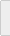HomeResourcesDoing a symetrical outer join with CASE

Howard,

I want to display sales summaries by vendor for this year and the previous year in an Active Server Page.  I know how to do this in a program, but I want to do it in a single result set. I tried joining the statements together, (left outer join), but sometimes there are no sales, (yet), for this year so I do not retrieve any records for the previous year.  I would really like to see a single record that contains all of the data for a vendor, both this year and last but includes the previous years data. Can you help? - Sam

Dear Sam,

Actually, what you really want is called a union or a symmetric outer join.  The union would actually be two statements, statement one with the outer join and statement two containing only records from last year that have no corresponding match in this year. By making a union, you would achieve a set that contains all results from both.  However, unions look really ugly and it still would not be in �one� record, so here is a prettier version of your statement that uses stupid case tricks:

Your statement alluded to a table that contained columns called vendor, yr, mo, sales and cost.  With those fields in mind, I constructed the following statement:

```SELECT VENDOR,
DOUBLE(CASE WHEN yr=2002 THEN SALES
ELSE 0 END) AS YTD,
DOUBLE(CASE WHEN yr=2001 THEN SALES
ELSE 0 END) AS PYYTD,
DOUBLE(CASE WHEN yr=2002 THEN SALES-COST
ELSE 0 END) AS YTDGP,
DOUBLE(CASE WHEN yr=2001 THEN SALES-COST
ELSE 0 END) AS PYYTDGP
FROM VENDORSALES
WHERE ((mo<=4 AND yr=2002) OR (mo<=4 AND yr=2001))```

Now, note the second field that I am selecting. It is an expression that evaluates as the following: when the YR field equals the 2002 then return the SALES field else return 0.  For the following example data

Vendor Yr Sales Cost
ACME 2002 110 70
ACME 2001 200 190

The select statement would return the following fields:

VENDOR

YTD

PYYTD

YTDGP

PYYTDGP

ACME

110

0

70

0

ACME

0

200

0

10

So, you are close to what you want because the resultant statement will retrieve all records where the yr is in 2002 or 2001 and the month is less than 4, and we avoided joining the table to itself so we are assured of getting all records. But now we need to aggregate the data into a single row.  For this, we will use a device called a common table expression.  A common table expression is where you select from a select statement rather than selecting from a table. Here is your new statement

```SELECT VENDOR,
SUM(ytd) AS YTD, SUM(ytdgp) AS YTDGP,
(CASE WHEN SUM(ytd)>0 THEN
SUM(ytdgp)/SUM(ytd)*100 ELSE 0 END) AS YTDGPP,
SUM(PYYTD) AS PYYTD,
SUM(PYYTDGP) AS PYYTDGP,
(CASE WHEN SUM(pyytd)>0
THEN SUM(pyytdgp)/SUM(pyytd)*100
ELSE 0 END) AS pyYTDGPP,
(CASE WHEN SUM(ytdgp)>0 THEN
(SUM(ytdgp)-SUM(pyytdgp))/SUM(ytdgp)*100
ELSE 0 END) AS PCTCHG
FROM  (SELECT VENDOR,
DOUBLE(CASE WHEN yr=2002 THEN SALES
ELSE 0 END) AS YTD,
DOUBLE(CASE WHEN yr=2001 THEN SALES
ELSE 0 END) AS PYYTD,
DOUBLE(CASE WHEN yr=2002 THEN SALES-COST
ELSE 0 END) AS YTDGP,
DOUBLE(CASE WHEN yr=2001 THEN SALES-COST
ELSE 0 END) AS PYYTDGP
FROM VENDORSALES
WHERE ((mo<=4 AND yr=2002) OR (mo<=4 AND yr=2001))
) AS RAWDATA
GROUP BY VENDOR ORDER BY 3 DESC```

Note that in this statement, I added a few goodies.  First, the outer statement selects from the RAWDATA table, which is just a name that I gave the select statement. In this manner, the AS/400 treats the select statement as if was a virtual table, (view), which disappears as soon as the run of my select statement is complete.  Second, I added fields to calculate a gross profit percentage and also added a final field to calculate the percent change in gross profit between last year and this year. The resulting output should look something like this:

Vendor

YTD

YTDGP

YTDGPP

PYYTD

PYYTDGP

PYYTDGPP

PCTCHG

ACME

110

70

63.63

200

190

95%

-67.2%

JONES

230

101

43.91

209

107

51.1%

-5.94

Of course, the nice thing about the query is that since you are doing each of the calculations in the query itself you are free to order by the calculations or the vendor field.  Adding ORDER BY 2 DESC would order the results by year to date gross profit descending whereas ORDER BY 8 would order the result set by Percent Change ascending.  Common table expressions are a really cool way to create virtual columns or do aggregates. Next week, I take this query even further and show you how to use parameters to specify the values in your case statement. You can find out more about common table expression and parameters by going to www.sqlhting.com or buying my book, iSeries and AS/400 SQL at Work